### Arithmetic Progressions Class 10th Mathematics CBSE Solution

##### Question 1.Which term of the AP: 121, 117, 113, . . ., isits first negative term?[Hint: Find n for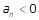]Answer: Here, first term, a = 121common difference, d = a2 - a1 = 117 - 121 = -4Let the first negative term be 'an'.Also, we know that, nth term of an AP is given byan = a + (n - 1)dWe have to find least value of n, such thatan < 0⇒ a + (n - 1)d < 0⇒ 121 + (n - 1)(-4) < 0⇒ 121 - 4(n - 1) < 0⇒ 4(n - 1) > 121⇒ 4n - 4 > 121⇒ 4n > 125⇒ n > 31.25Therefore, n is 32 [least positive integer greater than 31.25 is 32]Hence, the 32nd term of AP is first negative term.Also, a32 = a + 31d = 121 + 31(-4)= 121 - 124 = -3Question 2.The sum of the third and the seventh terms of an AP is 6 and their product is 8. Find the sum of first sixteen terms of the AP.Answer:To Find: S16Given: a3 + a7 = 6a3 x a7 = 8nth term of an AP is given by the formulaan = a + (n - 1) dwhere, an = nth term n = number of termd = common differenceSo now its given that sum of third and seventh term is 6, thus we need to find 3rd and 7th term first,a3 = a + 2da7 = a + 6dAs per question;a3 + a7 = 6So now,a + 2d + a + 6d = 62a + 8d = 6a + 4d = 3a = 3 – 4d ....................eq (i)Similarly,Product of third and seventh term is given as 8. So,(a + 2d)(a + 6d) = 8a2 + 6ad + 2ad + 12d2 = 8 ..........................eq(ii)Substituting the value of a in equation (ii), we get;(3 – 4d)2 + 8(3 – 4d)d + 12d2 = 89 – 24d + 16d2 + 24d – 32d2 + 12d2 = 89 – 4d2 = 82d = 1d = ± 1/2Using the value of d in equation (1), we get;a = 3 – 4d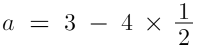Or, a = 3 – 2 = 1Sum of first 16 terms is calculated as follows: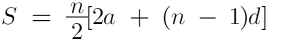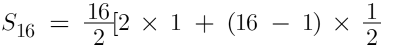S16 = 8[ 2 + (15/2)]= 4 x 19S16 = 76Thus, sum of first 16 terms of this AP is 76.Now by taking d = -1/2, we get,a = 3 - 4 (-1/2)a = 3 + 2 = 5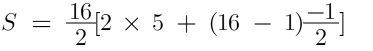S = 8[10 - 15/2]S = 4[20 - 15]S = 4 = 20So, another possible value of sum is 20. Question 3.A ladder has rungs 25 cm apart.(see Fig. 5.7). The rungs decrease uniformly in length from 45 cm at the bottom to 25 cm at the top. If the top and the bottom rungs are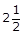m apart, what is the length of the wood required for the rungs?[Hint : Number of rungs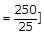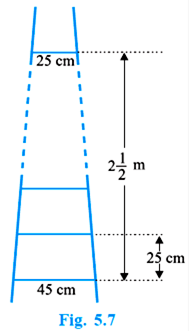Answer:Total distance between top and bottom rung = 2 m 50 cmDistance between any two rungs = 25 cmAnd it is also given that bottom most rungs is of 45 cm length and top most is of 25 cm length.As it is given that the length of rungs decrease uniformly, it will for an AP with a = 25, a11 = 45 and n = 11d can be calculated as follows:nth term of an AP is given byan = a + (n - 1)da11 = a + 10d45 = 25 + 10d10d = 45 – 25 = 20d = 2Total length of wood will be equal to the sum of 11 terms: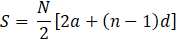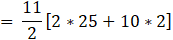= 11[ 25 + (10)]= 11 x 35= 385 cmTherefore, total wood required for rungs is equal to 385 m Question 4.The houses of a row are numbered consecutively from 1 to 49. Show that there is a valueof x such that the sum of the numbers of the houses preceding the house numbered x isequal to the sum of the numbers of the houses following it. Find this value of x.[Hint :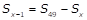]Answer:The AP in the above problem is1, 2, 3, - - - , 49With first term, a = 1Common difference, d = 1nth term of AP = a + (n - 1)dan = 1 + (n - 1)1an = n Suppose there exist a mth term such that, (m<49)Sum of first m - 1 terms of AP = Sum of terms following the mth termSum of first m - 1 terms of AP = Sum of whole AP - Sum of first m terms of APAs we know sum of first n terms of an AP is,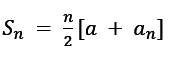if last term an is given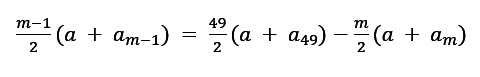(m - 1)(1 + m - 1) = 49(1 + 49) - m(1 + m) [using 1](m - 1)m = 2450 - m(1 + m)m2 - m = 2450 - m + m22m2 = 2450m2 = 1225m = 35 or m = - 35 [not possible as no of terms can't be negative.]and am = m = 35 [using 1]So, sum of no of houses preceding the house no 35 is equal to the sum of no of houses following the house no 35.Question 5.A small terrace at a football ground comprises of 15 steps each of which is 50 m long andbuilt of solid concrete.Each step has a rise of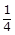m and a tread of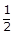m. (see Fig. 5.8). Calculate the total volumeof concrete required to build the terrace.[Hint : Volume of concrete required to build the first step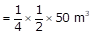]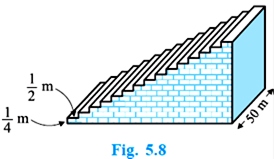Answer:Dimensions of 1st step = 50 m x 0.25 m x 0.5 mVolume of 1st step = 6.25 m3Dimensions of 2nd step = 50 m x 0.5 m x 0.5 mVolume of 2ndstep = 12.5 m3Dimensions of 3rdstep = 50 m x 0.75 m x 0.5 mVolume of 3rdstep = 18.75 m3Clearly, volumes of respective steps are in APNow, we have a = 6.25, d = 6.25 and n = 15Sum of 15 terms can be calculated as follows:S15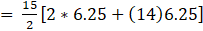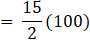= 750Hence, the volume of concrete will be 750m3

PDF FILE TO YOUR EMAIL IMMEDIATELY PURCHASE NOTES & PAPER SOLUTION. @ Rs. 50/- each (GST extra)

HINDI ENTIRE PAPER SOLUTION

MARATHI PAPER SOLUTION

SSC MATHS I PAPER SOLUTION

SSC MATHS II PAPER SOLUTION

SSC SCIENCE I PAPER SOLUTION

SSC SCIENCE II PAPER SOLUTION

SSC ENGLISH PAPER SOLUTION

SSC & HSC ENGLISH WRITING SKILL

HSC ACCOUNTS NOTES

HSC OCM NOTES

HSC ECONOMICS NOTES

HSC SECRETARIAL PRACTICE NOTES

# 2019 Board Paper Solution

HSC ENGLISH SET A 2019 21st February, 2019

HSC ENGLISH SET B 2019 21st February, 2019

HSC ENGLISH SET C 2019 21st February, 2019

HSC ENGLISH SET D 2019 21st February, 2019

SECRETARIAL PRACTICE (S.P) 2019 25th February, 2019

HSC XII PHYSICS 2019 25th February, 2019

CHEMISTRY XII HSC SOLUTION 27th, February, 2019

OCM PAPER SOLUTION 2019 27th, February, 2019

HSC MATHS PAPER SOLUTION COMMERCE, 2nd March, 2019

HSC MATHS PAPER SOLUTION SCIENCE 2nd, March, 2019

SSC ENGLISH STD 10 5TH MARCH, 2019.

HSC XII ACCOUNTS 2019 6th March, 2019

HSC XII BIOLOGY 2019 6TH March, 2019

HSC XII ECONOMICS 9Th March 2019

SSC Maths I March 2019 Solution 10th Standard11th, March, 2019

SSC MATHS II MARCH 2019 SOLUTION 10TH STD.13th March, 2019

SSC SCIENCE I MARCH 2019 SOLUTION 10TH STD. 15th March, 2019.

SSC SCIENCE II MARCH 2019 SOLUTION 10TH STD. 18th March, 2019.

SSC SOCIAL SCIENCE I MARCH 2019 SOLUTION20th March, 2019

SSC SOCIAL SCIENCE II MARCH 2019 SOLUTION, 22nd March, 2019

XII CBSE - BOARD - MARCH - 2019 ENGLISH - QP + SOLUTIONS, 2nd March, 2019

# HSCMaharashtraBoardPapers2020

(Std 12th English Medium)

HSC ECONOMICS MARCH 2020

HSC OCM MARCH 2020

HSC ACCOUNTS MARCH 2020

HSC S.P. MARCH 2020

HSC ENGLISH MARCH 2020

HSC HINDI MARCH 2020

HSC MARATHI MARCH 2020

HSC MATHS MARCH 2020

# SSCMaharashtraBoardPapers2020

(Std 10th English Medium)

English MARCH 2020

HindI MARCH 2020

Hindi (Composite) MARCH 2020

Marathi MARCH 2020

Mathematics (Paper 1) MARCH 2020

Mathematics (Paper 2) MARCH 2020

Sanskrit MARCH 2020

Sanskrit (Composite) MARCH 2020

Science (Paper 1) MARCH 2020

Science (Paper 2)

Geography Model Set 1 2020-2021

MUST REMEMBER THINGS on the day of Exam

Are you prepared? for English Grammar in Board Exam.

Paper Presentation In Board Exam

How to Score Good Marks in SSC Board Exams

Tips To Score More Than 90% Marks In 12th Board Exam

How to write English exams?

How to prepare for board exam when less time is left

How to memorise what you learn for board exam

No. 1 Simple Hack, you can try out, in preparing for Board Exam

How to Study for CBSE Class 10 Board Exams Subject Wise Tips?

JEE Main 2020 Registration Process – Exam Pattern & Important Dates

NEET UG 2020 Registration Process Exam Pattern & Important Dates

How can One Prepare for two Competitive Exams at the same time?

8 Proven Tips to Handle Anxiety before Exams!